NEET  >  Previous Year Questions: Rotational Motion

# Previous Year Questions: Rotational Motion

Test Description

## 22 Questions MCQ Test Physics Class 11 | Previous Year Questions: Rotational Motion

Previous Year Questions: Rotational Motion for NEET 2023 is part of Physics Class 11 preparation. The Previous Year Questions: Rotational Motion questions and answers have been prepared according to the NEET exam syllabus.The Previous Year Questions: Rotational Motion MCQs are made for NEET 2023 Exam. Find important definitions, questions, notes, meanings, examples, exercises, MCQs and online tests for Previous Year Questions: Rotational Motion below.
Solutions of Previous Year Questions: Rotational Motion questions in English are available as part of our Physics Class 11 for NEET & Previous Year Questions: Rotational Motion solutions in Hindi for Physics Class 11 course. Download more important topics, notes, lectures and mock test series for NEET Exam by signing up for free. Attempt Previous Year Questions: Rotational Motion | 22 questions in 22 minutes | Mock test for NEET preparation | Free important questions MCQ to study Physics Class 11 for NEET Exam | Download free PDF with solutions
 1 Crore+ students have signed up on EduRev. Have you?
Previous Year Questions: Rotational Motion - Question 1

### Three particles A, B and C, each of mass m, are connected to each other by three massless rigid rods to form a rigid, equilateral triangular body of side l. This body is placed on a horizontal frictionless table (x-y plane) and is hinged to it at the point A so that it can move without friction about the vertical axis through A (see figure). The body is set into rotational motion on the table about A with a constant angular velocity w.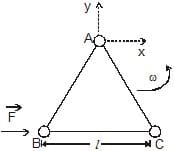[JEE'(Scr) 2002] (a) Find the magnitude of the horizontal force exerted by the hinge on the body (b) At time T, when the side BC is parallel to the x-axis, a force F is applied on B along BC (as shown). Obtain the x-component and the y-component of the force exterted by the hinge on the body, immediately after time T.

Detailed Solution for Previous Year Questions: Rotational Motion - Question 1

ATQ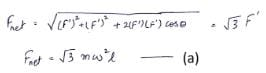Now,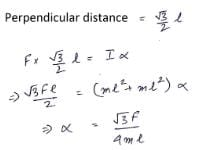So,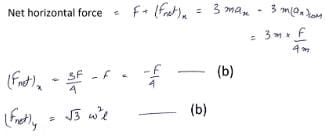Hence the answers should be changed to the ones in the solution marked as (a) and (b)

Previous Year Questions: Rotational Motion - Question 2

### A particle is moving in a horizontal uniform circular motion. The angular momentum of the particle is conserved about the point :   [JEE'(Scr) 2003]

Detailed Solution for Previous Year Questions: Rotational Motion - Question 2

The net force acting on a particle undergoing uniform circular motion is centripetal force which always passes through the centre of the circle. The torque due to this force about the centre is zero, therefore,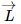is conserved about O.

Previous Year Questions: Rotational Motion - Question 3

### Two particles each of mass M are connected by a massless rod of length l. The rod is lying on the smooth surface. If one of the particle is given an impulse MV as shown in the figure then angular velocity of the rod would be [JEE'(Scr) 2003]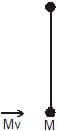Detailed Solution for Previous Year Questions: Rotational Motion - Question 3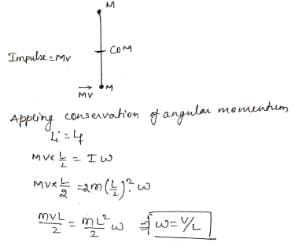Previous Year Questions: Rotational Motion - Question 4

A disc is rolling (without slipping) on a horizontal surface. C is its center and Q and P are two points equidistant from C. Let Vp, VQ and VC be the magnitude of velocities of points P, Q and C respectively, then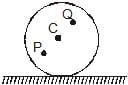[JEE'(Scr) 2004]

Previous Year Questions: Rotational Motion - Question 5

A child is standing with folded hands at the centre of a platform rotating about its central axis. The kinetic energy of the system is K. The child now stretches his arms so that the moment of inertia of the system doubles. The kinetic energy of the system now is

[JEE'(Scr) 2004]

Previous Year Questions: Rotational Motion - Question 6

A block of mass m is held fixed against a wall by a applying a hor izontal force F. Which of the following option is incorrect :

[JEE'(Scr) 2005]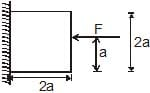Detailed Solution for Previous Year Questions: Rotational Motion - Question 6

A. It is correct.
B. As F passes through centre torque will be 0.
C. Since the frictional force produces a torque, so, to balance it out normal force will also produce a torque.
D. It is also correct.

Previous Year Questions: Rotational Motion - Question 7

A disc has mass 9M. A hole of radius R/3 is cut from it as shown in the figure. The moment of inertia of remaining part about an axis passing through the centre `O' of the disc and perpendicular to the plane of the disc is :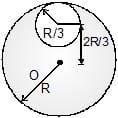[JEE'(Scr) 2005]

Detailed Solution for Previous Year Questions: Rotational Motion - Question 7

The moment of inertia of complete disc about a perpendicular axis passing through center O is,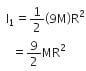The mass of cut out disc if radius R/3 is,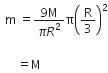Now, using the theorem of parallel axis, the moment of inertia of cut out disc about the perpendicular axis passing through center O is,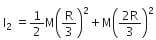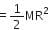The moment of inertia of residue disc is,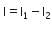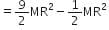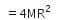Previous Year Questions: Rotational Motion - Question 8

A particle moves in circular path with decreasing speed. Which of the following is correct

Detailed Solution for Previous Year Questions: Rotational Motion - Question 8

Only the direction of L is constant because L = m v x r

Previous Year Questions: Rotational Motion - Question 9

A solid sphere of mass M, radius R and having moment of inertia about an axis passing through the centre of mass as I, is recast into a disc of thickness t, whose moment of inertia about an axis passing through its edge and perpendicular to its plane remains I. Then, radius of the disc will be

[JEE' 2006]

*Multiple options can be correct
Previous Year Questions: Rotational Motion - Question 10

A solid cylinder of mass m and radius r is rolling on a rough inclined plane of inclination q. The coefficient of friction between the cylinder and incline is m. Then

[JEE' 2006]

Detailed Solution for Previous Year Questions: Rotational Motion - Question 10

Fnet = mgsinθ – f
And Torque = F x R
So, if θ is decreased then torque is reduced and resultantly angular acceleration is reduced. Clearly, friction is opposing the translation and supports rotation.

*Multiple options can be correct
Previous Year Questions: Rotational Motion - Question 11

A ball moves over a fixed track as shown in the figure. From A to B the ball rolls without slipping. Surface BC is frictionless. KA, KB and KC are kinetic energies of the ball at A, B and C, respe0ctively. Then

[JEE' 2006]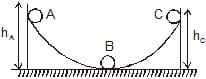Detailed Solution for Previous Year Questions: Rotational Motion - Question 11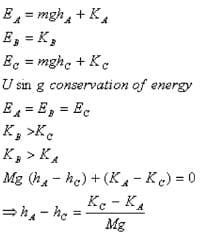Previous Year Questions: Rotational Motion - Question 12

There is a rectangular plate of mass M kg of dimensions (a × b). The plate is held in horizontal position by striking n small balls each of mass m per unit area per unit time. These are striking in the shaded half region of the plate. The balls are colliding elastically with velocity v. What is v ?

[JEE' 2006]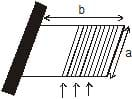It is given n = 100, M = 3 kg, m = 0.01 kg; b = 2 m, a = 1m; g = 10 m/s2.

Detailed Solution for Previous Year Questions: Rotational Motion - Question 12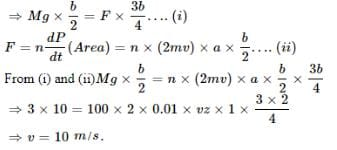Previous Year Questions: Rotational Motion - Question 13

Two discs A and B are mounted coaxially on a vertical axle. The discs have moments of inertia I and 2I respectively about the common axis. Disc A is imparted an initial angular velocity 2w using the entire potential energy of a spring compressed by a distance x1. Disc B is imparted an angular velocity w by a spring having the same spring constant and compressed by a distance x2. Both the discs rotate in the clockwise direction.

The ratio x1/x2 is

[JEE' 2007]

Previous Year Questions: Rotational Motion - Question 14

Two discs A and B are mounted coaxially on a vertical axle. The discs have moments of inertia I and 2I respectively about the common axis. Disc A is imparted an initial angular velocity 2w using the entire potential energy of a spring compressed by a distance x1. Disc B is imparted an angular velocity w by a spring having the same spring constant and compressed by a distance x2. Both the discs rotate in the clockwise direction.

When disc B is brought in contact with disc A, they acquire a common angular velocity in time t. The average frictional torque on one disc by the other during this period is

[JEE' 2007]

Previous Year Questions: Rotational Motion - Question 15

Two discs A and B are mounted coaxially on a vertical axle. The discs have moments of inertia I and 2I respectively about the common axis. Disc A is imparted an initial angular velocity 2w using the entire potential energy of a spring compressed by a distance x1. Disc B is imparted an angular velocity w by a spring having the same spring constant and compressed by a distance x2. Both the discs rotate in the clockwise direction.

The loss of kinetic energy during the above process is

[JEE' 2007]

Previous Year Questions: Rotational Motion - Question 16

A small object of uniform density rolls up a curved surface with an initial velocity v. It reaches up to a maximum height of 3v2 / (4g) with respect to the initial position. The object is

[JEE' 2007]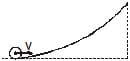Previous Year Questions: Rotational Motion - Question 17

STATEMENT-1 If there is no external torque on a body about its center of mass, then the velocity of the center of mass remains constant

because

STATEMENT-2 The linear momentum of an isolated system remains constant.

[JEE 2007]

Detailed Solution for Previous Year Questions: Rotational Motion - Question 17

The true statements are

1. If there is no external torque on a body about its center of mass, then the angular velocity of the center of mass remains constant
2. The linear momentum of an isolated system remains constant.

Previous Year Questions: Rotational Motion - Question 18

STATEMENT-1

Two cylinders, one hollow (metal) and the other solid (wood) with the same mass and identical dimensions are simultaneously allowed to roll without slipping down an inclined plane from the same height. The hollow cylinder will reach the bottom of the inclined plane first.

STATEMENT-2

By the principle of conservation of energy, the total kinetic energies of both the cylinders are identical when they reach the bottom of the incline.

[JEE-2008]

Previous Year Questions: Rotational Motion - Question 19

If the resultant of all the external forces acting on a system of particles is zero, then from an inertial frame, one can surely say that

[JEE 2009]

*Multiple options can be correct
Previous Year Questions: Rotational Motion - Question 20

A sphere is rolling without slipping on a fixed horizontal plane surface. In the figure is the point of contact, is the centre of the sphere and is its topmost point Then,

[JEE 2009]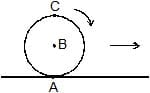Previous Year Questions: Rotational Motion - Question 21

A boy is pushing a ring of mass 2 kg and radius 0.5 m with a stick as shown in the figure. The stick applies a force of 2 N on the ring and rolls it without slipping with an acceleration of 0.3 m/s2. The coefficient of friction between the ground and ring is large enough that rolling always occurs and the coefficient of friction between the stick and the ring is (P/10). The value of P is?

[JEE 2011]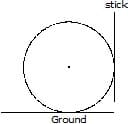Detailed Solution for Previous Year Questions: Rotational Motion - Question 21

Let the friction between ground and sphere be fg
Let the friction between stick and sphere be fs​
Let the N be the normal reaction between sphere and stick
By 2nd law of motion
N−fg​= ma and N= 2Newton,
fg​= 1.4Newton
Torque about center is written by τ= Iα and τ= F x r
Hence (fg​−fs) r=Iα
Also, a= αr and I=mr2
On solving fs​=0.8=μN
μ= 0.4= 4/10 therefore P= 4.

Previous Year Questions: Rotational Motion - Question 22

A thin uniform rod, pivoted at O is rotating in the horizontal plane with constant angular speed w, as shown in the figure. At time t = 0, small insect starts from O and moves with constant speed n with respect to the rod towards the other end. it reaches the end of the rod at t = T and stops. The angular speed of the system remains w throughout. The magnitude of the torque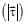on the system about O, as a function of time is best represented by which plot?

[JEE 2012]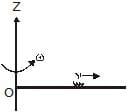Detailed Solution for Previous Year Questions: Rotational Motion - Question 22## Physics Class 11

127 videos|464 docs|210 tests
 Use Code STAYHOME200 and get INR 200 additional OFF Use Coupon Code
Information about Previous Year Questions: Rotational Motion Page
In this test you can find the Exam questions for Previous Year Questions: Rotational Motion solved & explained in the simplest way possible. Besides giving Questions and answers for Previous Year Questions: Rotational Motion , EduRev gives you an ample number of Online tests for practice

## Physics Class 11

127 videos|464 docs|210 tests

### How to Prepare for NEET

Read our guide to prepare for NEET which is created by Toppers & the best Teachers